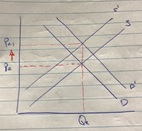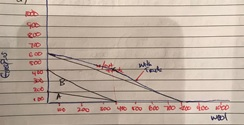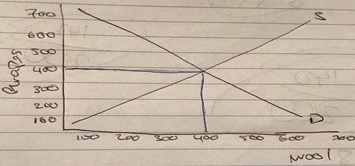# ECON 130 Tutorial 6 Question

Econ 130 Tutorial 6

MC1. Which of the following would result in an increase in equilibrium price and an ambiguous change in equilibrium quantity?

1. A decrease in supply and an increase in demand will result to the price equilibrium to shift upwards and the demand to increase because of the shortage.MC2. Which statement is incorrect? D. Country A does not has a comparative advantage in producing beer. It is country B that has the comparative advantage in producing beer.

MC3. Shares have a higher long-run average return than bonds. This is because

2. Because bonds are guaranteed and shares are not guaranteed which makes it riskier. The difference between stocksand bondsis that stocks are shares in the ownership of a business, while bonds are a form of debt that the issuing entity promises to repay at some point in the future.

Q1. 1. Draw a diagram to show an aggregate production possibility frontier without trade and one with trade. With reference to your diagram, explain which country would export each good and why the two countries might benefit from such trade.Slope of the curve when the trade represents the opportunity cost of the country producing the good. Country A, for every grapes it gives up 2 wool. That makes the opportunity cost of country A 0.5. Country B, for every grape it gives up 1 wool and for every wool it gives up 1 grape therefore country B has the competitive advantage over country A in grapes.

3. Noting the assumption above about complete specialisation, find the equilibrium relative price of grapes. Find the consumption per household (for both goods) for country A, and for country B.Qwa = 400, Qgb = 400 is where the equilibrium is. P=1, country A is better off while country B no better or worse. P=2, country A no better or worse while country B is better off. Each country would get half of goods (200 of each goods) with 400 household consume 0.5 of each unit.

4. Do the households in each country benefit from the free trade deal? Discuss

Trade should always benefit both countries. At the very least, one should be better off the other should be no worse off. Country A is better off from the trade country B will be no worse off.

Q2. Calculate the Net Present Value (NPV) of profit for Firm A in each of the four scenarios.

The formulae or calculation to calculate the Net Present Value of profit is as follows: PV= Profit/(1.r)^1.

1. -30/(1.1)^1 = -27. 60/(1.1)^2 = 49.6. 10/(1.1)^3 = 45. -27+49.6+45 = 67.5 PV -30/(1.1)^1 = -27. 10/(1.1)^2 = 8.3. 0/(1.1)^3 = 0. -27+8.3+0 = 35.3 PV

40/(1.1)^1 = 30. 15/(1.1)^2 = 12.4. 0/(1.1)^3 = 0. 30+12.4+0 = 42.4 PV

10/(1.1)^1 = 9. 10/(1.1)^2 = 8.2. 0/(1.1)^3 = 0. 9+8.2+0 = 17.3 PV

These are the calculation of the net present value.